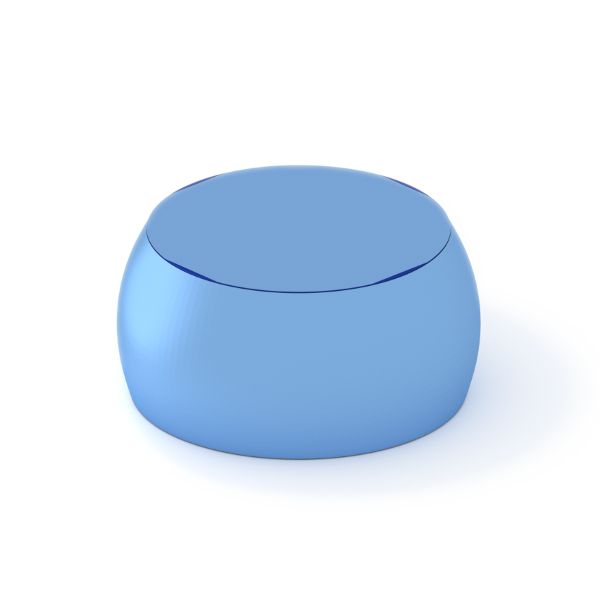### TruncatedSphere

A spherical segment, obtained from a spherical ball by two parallel cuts.#### Constructor

TruncatedSphere(R, H, dh)


Parameters:

• H, untruncated height (height before top removal)
• dh, top removal

Constraint:

$dh < H \le 2R$

#### Usage

As for any other Form factor.

#### Implementation

Class TruncatedSphere inherits from the interface class IFormFactor .

Computation involves numerical integration in vertical direction,

$$F(\mathbf{q})=2\pi \exp[iq_z(H-R)] \int_{R-H}^{R-dh} \text{d}z \space R_z^2 \frac{J_1(q_{||}R_z)}{q_{||}R_z} \exp(iq_z z),$$

with the notation

$$q_{||} := \sqrt{q_x^2 + q_y^2}, \quad R_z:=\sqrt{R^2-z^2}$$

Volume has been validated against $$V=\dfrac{\pi}{3} [ 3R(H^2-dh^2) + dh^3 -H^3 ].$$

More general:

More special:

• Sphere, if $H=2R, \space dh=0.$

#### Example

Scattering by uncorrelated, oriented truncated spheres for horizontal incidence. Rotation around $y$ axis:Generated by Examples/ff/TruncatedSphere.py .

#### History

Agrees with the IsGISAXS form factor “Sphere” [manual, Eq. 2.32] and “Truncated sphere” [Renaud 2009, Eq. 228]. It is not “Truncated sphere” of FitGISAXS, which is without top removal [Babonneau 2013].